Analogue Report ToolboxThis MATLAB toolbox [zip] brings together models and methods from our lab for analyzing behavioural responses on analogue report (or “delayed estimation”) tasks, where both stimuli and responses are chosen from a continuous parameter space. It implements three main methods:

1. Neural Resource model (Stochastic sampling). Code to fit a detailed model of population coding: either the original 1D (report dimension only) model with a fixed per-item swap probability, or the full 2D version implementing variability in both cue and report dimensions. This model captures effects of set size on the basis of sharing a fixed amount of population activity between items in memory; to constrain model parameters it is recommended to fit this model to data from multiple set sizes. The code takes advantage of the equivalence between population coding and stochastic sampling to optimize computations. Refs: Bays, J Neurosci (2014); Schneegans & Bays, J Neurosci (2017); Schneegans, Taylor & Bays, PNAS (2020).

2. Mixture model. This older code fits a descriptive model to data from a single set size. Responses are described by a probabilistic mixture of errors centred on the target feature, centred on one of the non-target features, or distributed uniformly across the response space. Refs: Bays, Catalao & Husain, J Vis (2009); Schneegans & Bays, Cortex (2016).

3. Non-parametric (NP) swap methods. The purpose of this code is to provide estimates of swap frequency and response-dimension variability while making the fewest possible assumptions about the parametric form of the error distribution. This method avoids biases inherent in the mixture model approach, but also requires more data than the parametric methods. Ref: Bays, Sci Rep (2016).

The code is released under a GNU General Public License: feel free to use and adapt these functions as you like, but credit should be given if they contribute to your work by citing appropriate references from the above. If you refer to this code directly, please also include the URL of this webpage, or our homepage bayslab.com.

Introduction

A typical example of an analogue report task is illustrated in Fig. 1. The response parameter space in this case is represented by a 'colour wheel'. On each trial a sample array is presented consisting of a set of items with colours chosen at random from the wheel. The number of items in the sample array is called the set size (here, 3). After a blank retention period, one of the preceding colours (the target) is indicated by a cue at the same location and the participant clicks on the wheel to indicate the colour they recall seeing at that location.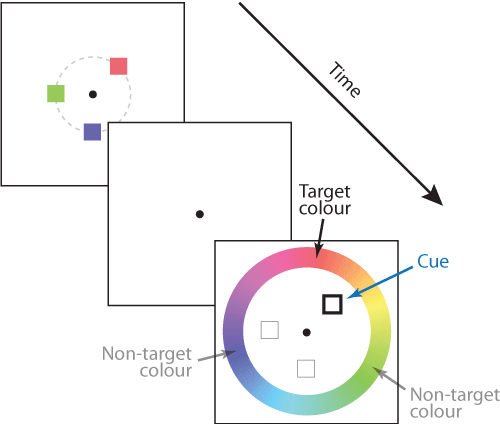Figure 1 | The colour report task.

An important aspect of tasks like this one is that accurate performance requires memory not only for colour (the report dimension) but also for location (the cue dimension). An observer with no memory for location would be equally likely to report any of the colours in the sample array. In this case the item locations are chosen from an invisible circle, so all the information in the sample array relevant to the task can be described as a set of points, one for each item, in a 2D space with one axis indicating a colour on the colour wheel and the other a radial location. This is illustrated in Fig. 2A.

Visual areas of the brain are known to represent these kind of stimulus features using population codes. Fig. 2B illustrates how a population of idealized neurons could encode the information in Fig. 2A. The colour of each pixel shows the activity level of neurons whose preferred stimulus (the pairing of colour and location that activates them most strongly) is indicated by the position of the pixel on x and y scales.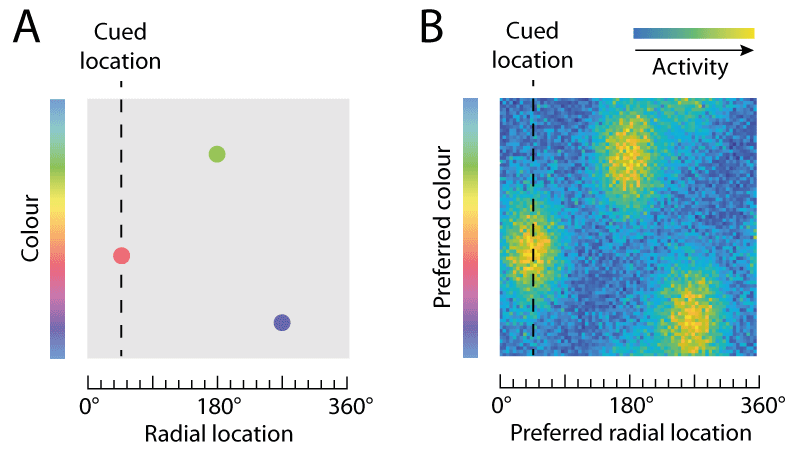Figure 2 | 2D and population representations of items shown in Fig. 1.

Variability in retrieving features of the sample array (e.g. due to the noise in population activity illustrated in Fig. 2B) affects responses in two ways. Variability in the cue dimension (here, location) means that the item matching the cue is sometimes misidentified, with the result that one of the non-target items is reported instead of the target; this is known as a swap error. Whichever item is chosen for report, variability in the report dimension (colour) causes random dispersion of responses around the true feature value that was present in the sample array. An illustrative probability distribution of responses for the example trial is shown in Fig. 3: most responses are distributed around the target colour (orange-red) but a minority are distributed around one or other of the non-target colours.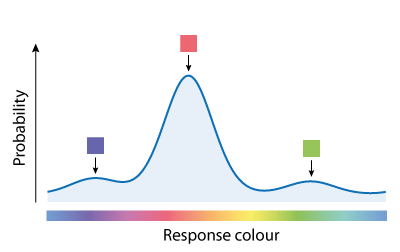Figure 3 | Probability distribution of responses on a single trial.
Circular parameters

The functions in this toolbox require inputs (stimulus feature and response values) to be specified in radians, in the range [−π, π]. Values coded in degrees can be converted to radians as follows:

X = wrap(X_deg/180*pi)

where the wrap function constrains the resulting response values to the required range. The wrap function can also be used when adding or subtracting circular values, e.g. the error on a trial can be calculated as the deviation between the response value X and the target value T:

E = wrap(X-T)

will ensure the resulting error measure is still in the range [−π, π].

There is the possibility of confusion with tasks testing recall of orientations. If the task involves reporting the remembered orientation of a bar, for example, the range of unique angles covers 180° (π radians) rather than 360° (2π radians). In this case, the orientation values need to be multiplied by 2 so that the range [−π, π] covers the unique angles only, e.g.

X = wrap(X_deg/90*pi)

In addition to the main methods, this toolbox contains a number of helper functions that may be useful for working with circular variables.

Neural Resource model (Stochastic sampling)

These functions can be used to fit a population coding model of working memory to behavioural data. It follows Schneegans, Taylor & Bays (PNAS, 2020) which presented a re-conceptualization of the Neural Resource model (Bays, J Neurosci, 2014; Schneegans & Bays, J Neurosci, 2017) as stochastic sampling. These MATLAB functions provide a user-friendly method to generate predictions from the model and fit it to data. If you were looking for the data from the PNAS paper and code to reproduce the figures, they can be found on OSF [here].

Theory. Continuous visual features, such as colour, orientation or location, are encoded in neural activity using population codes. A large number of neurons are sensitive to each feature, but vary in their preferred values in such a way that their tuning curves cover the space of possible feature values (Fig. 4, top). The Neural Resource model proposed that recall errors in working memory tasks result from decoding a feature value from spiking activity in such a neural population. To begin with, we restrict our modelling to only the representation of the report feature dimension. For simplicity, we assume an idealized population of neurons with identical tuning functions described by a Gaussian function, with preferred values densely and evenly distributed over a one-dimensional feature space. These neurons generate discrete spikes through a Poisson process. The model has two free parameters, namely the precision (inverse variance) of the Gaussian tuning curves, ω1, and the mean number of spikes generated by the population in a fixed decoding interval, γ.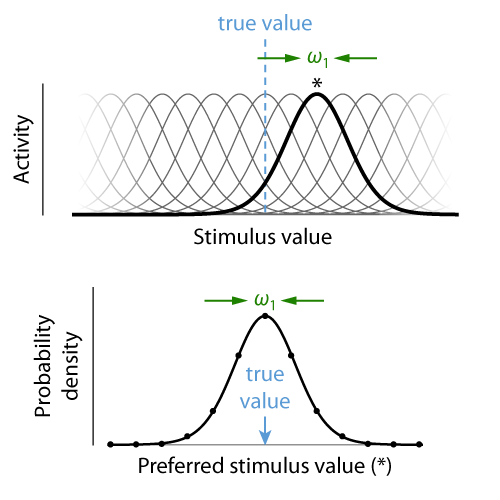Figure 4 | Tuning curves of a population of neurons with tuning precision ω1 (top) and corresponding probability distribution over the preferred feature value of the neuron generating each spike (bottom).

If we associate each spike in the population with the preferred value of the neuron that generated it, we can interpret the spiking activity as drawing random samples from a probability distribution that has the same shape as the neural tuning curves and is centred on the true stimulus feature encoded in the population activity (Fig. 4, bottom). The more spikes, or samples, that are generated, the more precisely we can estimate this true stimulus feature. If the spikes from each neuron are generated through a Poisson process, the total number of spikes in the neural population is also a Poisson random variable. Moreover, if the tuning curves (and thus the distribution from which samples are drawn) are Gaussian, the distribution of decoding errors is likewise Gaussian, and its precision increases linearly with the number of samples. Thus, the distribution of recall errors can be described by a weighted mixture of Gaussian distributions, with weights following a Poisson distribution (Fig. 5). For circular feature spaces as typically used experimentally, this is only an approximation, but the discrepancy from the exact distribution is very small for the range of parameters typically found in fits to behavioral data. This idealized model also matches well to simulations of heterogeneous correlated neural populations that more closely represent the biological system.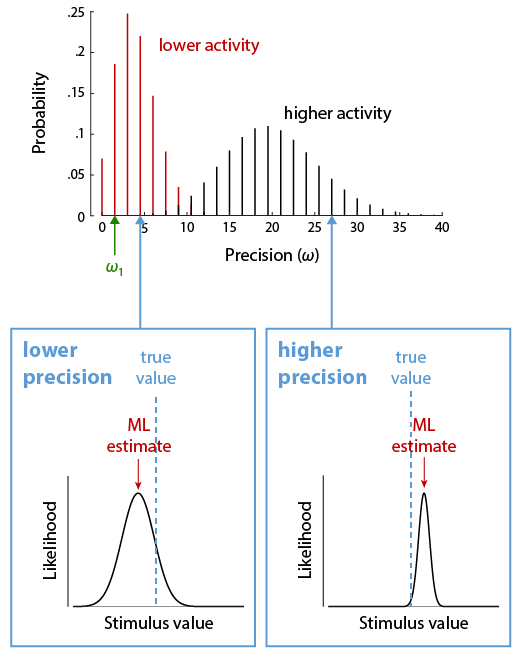Figure 5 | The distribution over decoding precision (defined here in terms of the width of the likelihood function, see insets) follows a Poisson distribution, scaled by the precision ω1 of the individual tuning functions.

Using the functions. Example code for fitting the Neural Resource model to behavioural data and plotting the results is provided in the file demoNeuralModel.m. Typically a separate maximum likelihood fit of the model parameters to the behavioural data of each subject is performed (e.g. using the Matlab function fminsearch). The key functions for this are described below.

The function

P = pNeuralModel(E, kappa, gamma)

computes the probability of obtaining response errors E when decoding the feature of a single item from a neural population with parameters kappa and gamma. The function implements both the exact error distribution in circular spaces (used by default) and the approximation, which is much faster to compute. An optional fourth parameter exactCircularMLE is used to switch between the two versions. The function can also be used for plotting the distribution of response errors in the model by providing a vector of evenly spaced values over the range [–π, π] as argument E.

In typical working memory experiments, multiple sample items are presented on each trial. The Neural Resource model assumes that the total neural activity encoding all items remains constant (is normalized), so that the mean number of spikes that contribute to the recall of each feature decreases with increasing number of items. In addition, when multiple items are stored simultaneously, swap errors (see Introduction) can occur in which a participant reports the feature of an item other than the target item identified by the cue. We first describe a function that models swap errors in a theory-neutral manner, as a fixed per-item probability of reporting each non-target item, before describing a more detailed approach based on conjunction coding of both cue and report feature dimensions.

The function

LL = llNeuralSwap(X, T, NT, nItems, kappa, gamma, pNT)

can be used to determine the log-likelihood of the Neural Resource model with specified parameters for a collection of trials with different set sizes. The column vectors X and T should contain the response feature value and the target feature in each trial, respectively. The matrix NT should contain in each row the feature values of all non-target items in each trial, and nItems should be the number of items in each trial (NT may contain NaNs or arbitrary values for unused item positions in a trial). The parameters kappa and gamma are the concentration of the tuning curves and the total mean firing rate in the neural population, respectively, and pNT is a fixed probability of selecting each non-target item for response generation in each trial. The function llNeuralSwap calls pNeuralModel to obtain the response distributions around each item, and like that function can be switched between the exact method and the approximation via an optional last argument in the function call.

Memory for feature conjunctions. The Neural Resource model as described above makes the simplifying assumption that only the feature that is to be reported needs to be decoded from neural activity. But, as described in the Introduction, to actually solve a delayed reproduction task, the neural system needs to encode the specific combinations of reported feature and cue feature (e.g. between the colour of a stimulus and its location in a sample array, if location is used to cue which item should be reported). These feature combinations can be encoded in the form of a conjunctive population code, in which each neuron's response is determined by its tuning curves in two different feature dimensions. The following functions extend the Neural Resource model to use such conjunctive representations.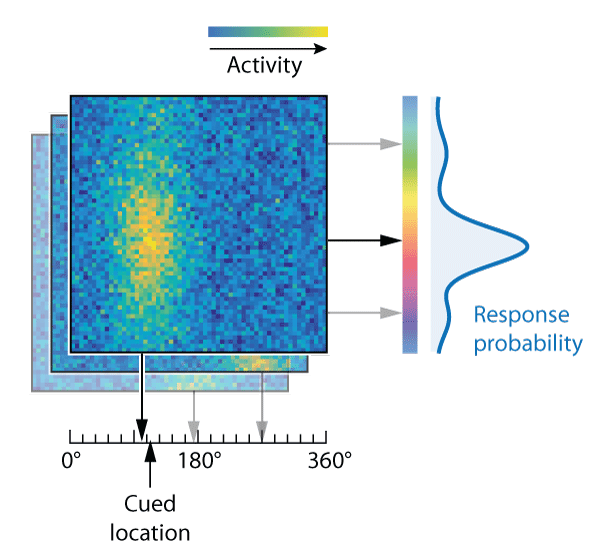Figure 6 | Decoding of stimulus features from spikes in a conjunctive population code, illustrating the probability distribution of colour responses (right) based on a location cue (bottom) for an example 3-item trial.

This neural binding model assumes that both cue and report feature values of all memorized items are decoded from the conjunctive population representation at the time when a cue is presented (Fig. 6). The item whose decoded cue feature value is closest to the given cue is selected, and its decoded report feature value is produced as a response. In this account, swap errors arise due to noise in decoding the cue features of memorized items, and depend on the similarity between different items in the cue feature dimension (Fig. 6, right). The original publication of this model (Schneegans & Bays, J Neurosci, 2017) used a continuous (Gaussian noise) approximation to discrete neural spiking, but here we provide a new implementation that directly extends the mechanism of the Neural Resource model. The model still makes the simplifying assumption that the neural activity associated with each item can be decoded independently of other items (as depicted by layers in Fig. 6), and it requires that cue features, like report features, are drawn from a 1-D circular space.

The function

P = pNeuralBinding(X, MR, C, MC, gamma, kappaR, kappaC)

determines the probability of obtaining response values X from the neural binding model for the given item features and model parameters. The matrices MR and MC specify the report and cue features of all the items (in the format [trials × items]), and the column vector C specifies for each trial the single feature value used as a cue (typically that will be the cue feature value of the target item, but the model also allows other values to be used). The following arguments provide the total spike rate, gamma, and the tuning curve concentrations, kappaR and kappaC, for the report and cue feature dimension, respectively. Like in the previous functions, switching between exact computations for circular space and the approximation is possible via an optional final argument.

The script demoNeuralBinding.m demonstrates how the above function can be used to fit the neural binding model to behavioral data. Also provided are functions to plot response distributions, and to simulate responses from the model. Note that the function pNeuralBinding assumes that the number of items is fixed across all trials (equal to the number of columns in matrices MR and MC); to fit data across multiple set sizes, multiple calls of the function need to be combined in the optimization (as illustrated in demoNeuralModel.m for the one-dimensional Neural Resource model).

Mixture model

The following functions can be used for fitting data from analogue report tasks with a 3-component mixture model, as first described in Bays, Catalao & Husain, J Vis (2009).

This method can be useful for evaluating swap errors based on relatively small numbers of trials at a single set size. However we urge caution in interpreting the other outputs: there is good reason to doubt the assumption (inherited from the precursor 2-component mixture model of Zhang & Luck, 2008, rather than theoretically motivated) that errors can be decomposed into normal and uniform components, and as a result one should beware the simplistic interpretation of the pU parameter as “guess frequency” or K as “memory precision” (see Taylor & Bays, Psych Rev, 2020). Also, the pN parameter has a tendency to underestimate swap frequency in comparison to more theory-neutral (but data-hungry) non-parametric methods (see below).

This descriptive model aims to capture analogue report performance as reflecting a probabilistic mixture of three kinds of response: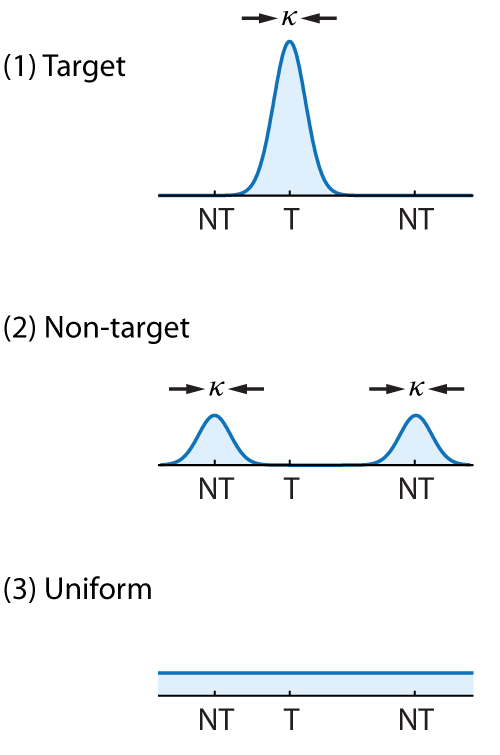Figure 7 | Mixture model components

(1) Target responses − the observer correctly reports the feature value (e.g. colour) of the cued item, with some variability,

(2) Non-target responses − the observer mistakenly reports the feature value of one of the other, uncued items held in memory with the same variability,

(3) Uniform responses − the observer generates a random response unrelated to either cued or uncued items.

Each of these components has a corresponding probability density function, illustrated in Fig. 7 for a 3-item sample array (one target value, T, and two non-target values, NT).

The contribution made by each of these components to the overall response distribution can be estimated with the function mixtureFit. The function uses an Expectation Maximization algorithm, with a range of initial parameter values, to efficiently fit the mixture model described above to data. Usage is as follows:

[B LL W] = mixtureFit(X, T, NT)

where X is an (n×1) vector of responses, T is an (n×1) vector of target values, and NT is an (n×m) matrix of non-target values.

Maximum likelihood estimates of the parameters of the mixture model are returned in B, a row vector of form [K pT pN pU]. K is the concentration parameter of the Von Mises distribution describing response variability, and [pT pN pU] are mixture parameters indicating the estimated probability of target, non-target and uniform responses, respectively (summing to 1). Optional output LL returns the log likelihood of the fitted model.

Optional output W is an (n×3) matrix of trial-by-trial posterior probabilities that responses are drawn from each of the three mixture components, as described in Schneegans & Bays, Cortex (2016). Each row corresponds to a separate trial and is of the form [wT wN wU], corresponding to the probability that the response on that trial came from the target, non-target or uniform response distributions, respectively.

If the input NT is omitted, the function will fit a mixture of target and uniform components only. Note that the fitting function is unlikely to provide reliable parameter estimates for n < 30 trials, and may fail altogether for small n.

Conversion between the Von Mises concentration parameter K and circular standard deviation is achieved with the functions:

sd = k2sd(K)
K = sd2k(sd)

Non-parametric (NP) swap estimation

The following functions provide non-parametric methods for estimating and excluding the influence of swap errors in analogue report tasks, as described in Bays, Sci Rep (2016).

The mixture model approach (above) to estimating the frequency of swap errors relies on specifying a model of variability in the report dimension (i.e. as a mixture of normal and uniform distributions). The NP method does not make any assumptions about this distribution. The function NP_alpha estimates the frequency with which different stimuli are reported. It can be used to estimate swap frequency as follows:

A = NP_alpha(X,T)

The input X is an (n×1) vector of responses; input T is an (n×m) matrix of report-dimension stimulus values (the first column should contain target values, the remaining columns non-target values). Output A is a (1×m) vector of mixture parameters summing to 1. The swap frequency can be obtained by summing the values in A corresponding to non-target inputs (all but the first value), or equivalently as one minus the first value in A (the target frequency).

Swap errors appear randomly-distributed relative to the target feature value, and so distort the "true" distribution of errors in the report feature dimension. The function NP_pdf can be used to exclude the influence of swap errors and uncover the true distribution:

P = NP_pdf(X,T)

Inputs as above. By default the distribution is evaluated at 25 evenly-spaced points on the circle. Use NP_pdf(X,T,Y) to specify evaluation points.

Similarly, swap errors distort estimates of parameters of the distribution, such as standard deviation. The function NP_moment can be used to recover the true circular moments of the error distribution:

M = NP_moment(X,T,J)

returns the Jth circular moment. For example, the true circular standard deviation of errors in the report dimension can be estimated by:

sqrt(-2*log(abs(NP_moment(X,T,1)))

By making fewer assumptions, the NP method has a substantial theoretical advantage over the older mixture model approach, which may be strongly biased due to incorrect specification of the distribution of errors, and in particular tends to underestimate swap frequency (see Bays, Sci Rep, 2016). However, the NP method requires more data to achieve a given level of variability in its estimates. As a result, I would not recommend using this method with n < 100 trials. Note also that for correct estimation using this method it is essential that stimuli are chosen independently and at random from the circular parameter space (e.g. no minimum separations between items).

The NP estimates occasionally fall outside the bounds of interpretable values, e.g. the range of probabilities [0, 1] for NP16_alpha. This is a desirable property: a bounded estimator, unless it is perfectly accurate, is necessarily biased in proximity to its bounds. However, a large out-of-bounds estimate can excessively influence group means. A compromise is to constrain the estimator beyond the true range: bounding individual observer estimates at [−1, 2] before calculating group means was found in simulations to provide a good balance between bias and variance for this estimator.

A demonstration of the use of these functions is included in the package (NP_demo.m).

Helper functions

In addition to those described above, the toolbox contains a number of additional functions that may be useful for analyzing data from analogue report tasks, and circular data more generally.

Correction for minimum feature distance. A simple heuristic to detect and visualize the presence of swap errors is to plot the histogram of response deviations from all non-target feature values. If responses are clustered around non-target features, this histogram should show a central peak, around deviations of zero (Bays, Catalao & Husain, J Vis, 2009). However, the interpretation of this histogram is more complicated if a minimum distance is enforced between the feature values in each trial, as is the case in some studies. To see this, consider the case that there is a large minimum distance between items, e.g. 30°, and all responses are clustered tightly around the actual target value (i.e., there are no swap errors). The histogram of response deviations from non-target features will then show a dip around zero, because all responses that are close to the target feature will necessarily have a distance of around 30° or more to all non-target features. This dip can obscure the presence of a central peak that would indicate the presence of swap errors.

We provide a method to correct for these effects of minimum feature distance, based on permutation of the non-target feature values (specified relative to the target feature) across trials (Schneegans & Bays, J Neurosci, 2017). Computing the response deviations from the permuted non-target features removes the signatures of actual swap errors, while still capturing the effects of minimum feature distance. This yields an expected histogram of non-target deviations in the absence of swap errors. Subtracting this from the histogram of actual non-target deviations yields a corrected function, which will be approximately uniform in the absence of swap errors, and display a central peak if swap errors are present.

The function

H = expectedNonTargetDeviation(X, T, NT, be)

computes the histogram of expected non-target deviations. Column vectors X and T are the response and target feature values, respectively, NT is the matrix of non-target feature values, and be is a vector of bin edges for the histogram. The resulting histogram is normalized as a probability density function. Note that it should be computed separately for each set size, with NT containing only the non-target feature values for that set size. An analogous correction is also implemented in the function errorDistributionsNeuralBinding.m for plotting the predictions of the neural binding model (see demoNeuralBinding.m).

Circular statistics. The toolbox contains functions to determine the circular mean (cmean), circular standard deviation (cstd) and resultant vector (cresultant) of a set of samples provided as input. Conversion between different measures of circular dispersion, namely circular standard deviation (sd), von Mises concentration (k) and precision defined as Fisher information (j) is implemented by the functions sd2k, k2sd, k2j, and j2k_interp (the latter uses interpolation for the conversion of Fisher Information to concentration, since no analytical solution exists).

The toolbox also implements probability density functions and cumulative distribution functions for the von Mises distribution (vonmisespdf and vonmisescdf) and the wrapped normal distribution (wrapnormpdf and wrapnormcdf). These distributions can both be viewed as circular equivalents to the normal (or Gaussian) distribution in Euclidean space, although neither possesses the full range of properties that make the normal distribution unique.

Random numbers. Random numbers drawn from a von Mises distribution with specified mean and concentration can be generated using the function randvonmises. The function randFromPdf allows drawing random numbers from a piecewise linear distribution that can be used to approximate arbitrary probability distributions. The function randMinDistance provides an efficient way to draw random numbers with a minimum distance between them (e.g. as feature values in single trial in a delayed reproduction task), both in linear and circular feature spaces.

Others. The function wrap maps inputs outside the circular space defined by [–π, π] back onto that interval, so is useful when e.g. adding or subtracting circular variables. The function circspace provides a quick way to create evenly spaced sampling points in circular space, with the distance between the first and last point matching the distance between any other adjacent points. For a given matrix of feature values in the format [trials × items] used here, and a column vector of item indices, the function pickFromRows can be used to extract the feature value at the given index in each trial. It can also produce a matrix of feature values with the specified item in each trial removed.

References

Bays PM, Catalao RFG & Husain M. The precision of visual working memory is set by allocation of a shared resource. Journal of Vision 9(10): 7, 1-11 (2009) [PDF | HTML | DATA]

Bays PM. Noise in neural populations accounts for errors in working memory. Journal of Neuroscience 34(10): 3632–3645 (2014) [PDF | HTML | DATA]

Bays PM. Evaluating and excluding swap errors in analogue tests of working memory. Scientific Reports 6: 19203 (2016) [PDF | HTML]

Schneegans S & Bays PM. No fixed item limit in visuospatial working memory. Cortex 83: 181–193 (2016) [PDF+SI | HTML]

Schneegans S & Bays PM. Neural architecture for feature binding in visual working memory. Journal of Neuroscience 37(14): 3913–3925 (2017) [PDF | HTML | DATA]

Taylor & Bays. Theory of neural coding predicts an upper bound on estimates of memory variability. Psychological Review [Advance online publication]. doi:10.1037/rev0000189 (2020) [PDF | HTML | CODE+DATA]

Schneegans S, Taylor R & Bays PM. Stochastic sampling provides a unifying account of working memory limits. Proceedings of the National Academy of Sciences Aug 2020, 202004306 (2020) [PDF+SI | HTML | CODE+DATA]